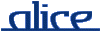# alice library manual.# The Vector structure

## ________ Synopsis ____________________________________________________

```    signature VECTOR
structure Vector : VECTOR
```

An extended version of the Standard ML Basis' Vector structure.

## ________ Import ______________________________________________________

Imported implicitly.

## ________ Interface ___________________________________________________

```    signature VECTOR =
sig
eqtype 'a vector
type   'a t = 'a vector

val maxLen :      int

val toList :      'a vector -> 'a list
val fromList :    'a list -> 'a vector
val tabulate :    int * (int -> 'a) -> 'a vector

val length :      'a vector -> int
val sub :         'a vector * int -> 'a
val update :      'a vector * int * 'a -> 'a vector
val concat :      'a vector list -> 'a vector
val rev :         'a vector -> 'a vector

val app :         ('a -> unit) -> 'a vector -> unit
val appr :        ('a -> unit) -> 'a vector -> unit
val map :         ('a -> 'b) -> 'a vector -> 'b vector
val foldl :       ('a * 'b -> 'b) -> 'b -> 'a vector -> 'b
val foldr :       ('a * 'b -> 'b) -> 'b -> 'a vector -> 'b
val all :         ('a -> bool) -> 'a vector -> bool
val exists :      ('a -> bool) -> 'a vector -> bool
val find :        ('a -> bool) -> 'a vector -> 'a option

val appi :        (int * 'a -> unit) -> 'a vector -> unit
val appri :       (int * 'a -> unit) -> 'a vector -> unit
val mapi :        (int * 'a -> 'b) -> 'a vector -> 'b vector
val foldli :      (int * 'a * 'b -> 'b) -> 'b -> 'a vector -> 'b
val foldri :      (int * 'a * 'b -> 'b) -> 'b -> 'a vector -> 'b
val alli :        (int * 'a -> bool) -> 'a vector -> bool
val existsi :     (int * 'a -> bool) -> 'a vector -> bool
val findi :       (int * 'a -> bool) -> 'a vector -> (int * 'a) option

val contains :    ''a vector -> ''a -> bool
val notContains : ''a vector -> ''a -> bool

val equal :       ('a * 'a -> bool) -> 'a vector * 'a vector -> bool
val collate :     ('a * 'a -> order) -> 'a vector * 'a vector -> order

val isSorted :    ('a * 'a -> order) -> 'a vector -> bool
val sort :        ('a * 'a -> order) -> 'a vector -> 'a vector
end
```

## ________ Description _________________________________________________

Items not described here are as in the Standard ML Basis' Vector structure.

type t = vector

A local synonym for type vector.

toList vec

Creates a list of the elements of vec in order of increasing indices.

rev vec

Returns a vector that contains the elements of vec in reverse order.

appr f vec
appri f vec

Like app and appi, but apply f in right to left order (i.e., decreasing indices). The expression appr f vec is equivalent to:

`        appri (f o #2) vec`
alli f vec
existsi f vec

Indexed versions of the functions all and exists. The index of each element is passed to f as an additional argument. The following equivalences hold:

```        all f vec    = alli (f o #2) vec
exists f vec = existsi (f o #2) vec```
contains vec a

Returns true if the element a occurs in the vector vec; otherwise false.

notContains vec a

Returns true if the element a does not occur in the vector vec; otherwise false. Equivalent to not(contains vec a).

equal eq (vec1, vec2)

Creates an equality function on vectors given an equality on the element type.

isSorted f vec

Returns true iff vec is sorted with respect to the ordering function f.

sort f vec

Returns a new vector that contains the same elements as vec, but sorted with respect to the ordering function f. Sorting may be unstable with respect to equal elements.